Courses

# Ex 8.2 NCERT Solutions- Quadrilaterals Class 9 Notes | EduRev

## Mathematics (Maths) Class 9

Created by: Full Circle

## Class 9 : Ex 8.2 NCERT Solutions- Quadrilaterals Class 9 Notes | EduRev

The document Ex 8.2 NCERT Solutions- Quadrilaterals Class 9 Notes | EduRev is a part of the Class 9 Course Mathematics (Maths) Class 9.
All you need of Class 9 at this link: Class 9

Question 1. ABCD is a quadrilateral in which P, Q, R and S are midpoints of the sides AB, BC, CD and DA (see figure). AC is a diagonal.
Show that: (i) SR || AC and SR =
(1/2) AC
(ii) PQ = SR (iii) PQRS is a parallelogram.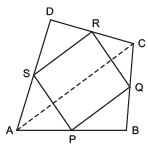Solution: We have P as the mid-point of AB, Q as the mid-point of BC, R as the mid-point of CD, S as the mid-point of DA, and AC as the diagonal of quadrilateral ABCD.

(i) To prove that SR =(1/2) AC and SR || AC.
In ΔACD, we have

S as the mid-point of AD, R as the mid-point of CD.
∵ The line segment joining the mid-point of any two sides of a triangle is parallel to the third side and half of it.
∴ SR = (1/2)AC and SR || AC

(ii) To prove that PQ = SR.
In ΔABC, we have P is the mid-point of AB, Q is the mid-point of BC.
∴ PQ = (1/2) AC            …(1)
Also, SR = (1/2) AC            [Proved]             …(2)
From (1) and (2), PQ = SR

(iii) To prove that PQRS is a parallelogram.
In ΔABC, P and Q are the mid-points of AB and BC.
∴ PQ = (1/2)AC and PQ || AC             ...(3)
In ΔACD, S and R are the mid-points of DA and CD.
∴ SR = (1/2)AC and SR || AC             …(4)
From (3) and (4), we get
PQ =(1/2)AC = SR and PQ || AC || SR
⇒ PQ = SR and PQ || SR
i.e. One pair of opposite sides in quadrilateral PQRS is equal and parallel.
∴ PQRS is a parallelogram.

Question 2. ABCD is a rhombus and P, Q, R and S are the mid-points of the sides AB, BC, CD and DA respectively.
Show that the quadrilateral PQRS is a rectangle.
Solution:
We have P as the mid-point of AB, Q as the midpoint of BC, R as the mid-point of CD, S as the mid-point of DS.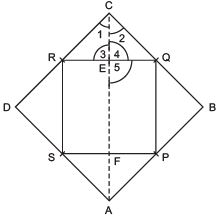We have to prove that PQRS is a rectangle.
Let us join AC.
∵ In ΔABC, P and Q are the mid-points of AB and BC.
∴ PQ =(1/2)AC and PQ || AC             …(1)
Also in ΔADC, R and S are the mid-points of CD and DA.
∴ SR = (1/2)AC and SR || AC

From (1) and (2), we get
PQ =(1/2) AC = SR and PQ || AC || SR
⇒ PQ = SR and PQ || SR
i.e. One pair of opposite sides of quadrilateral PQRS is equal and parallel.
∴ PQRS is a parallelogram.
Now, in ΔERC and ΔEQC, ∠1= ∠2            [∵ The diagonal of a rhombus bisects the opposite angles]
CR = CQ            [Each is equal to(1/2) of a side of rhombus]CE = CE [Common]
∴ ΔERC ≌ ΔEQC            [SAS criteria]
⇒ ∠3= ∠4[c.p.c.t.]
But ∠3 + ∠4 = 180º [Linear pair] ⇒ ∠3= ∠4 = 90°
But ∠5= ∠3            [Vertically opposite angles]
∴ ∠5 = 90º PQ || AC
⇒ PQ || EF
∴ PQEF is a quadrilateral having a pair of opposite sides parallel and one of the angles is 90º.
∴ PQEF is a rectangle.
⇒ ∠RQP = 90º
∴ One angle of parallelogram PQRS is 90º.
Thus, PQRS is a rectangle.

Question 3. ABCD is a rectangle and P, Q, R and S are the mid-points of the sides AB, BC, CD and DA respectively. Show that the quadrilateral PQRS is a rhombus.
Solution:
In a rectangle ABCD, P is the mid-point of AB, Q is the midpoint of BC, R is the mid-point of CD, S is the mid-point of DA AC is the diagonal.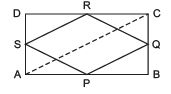Now, in ΔABC,
PQ =(1/2)AC and PQ || AC
[Mid-point theorem]             …(1)
Similarly, in ΔACD,
SR =(1/2)AC and SR || AC             …(2)
From (1) and (2), we get PQ = SR and PQ || SR Similarly, by joining BD,
we have PS = QR and PS || QR
i.e. Both pairs of opposite sides of quadrilateral PQRS are equal and parallel.
∴ PQRS is a parallelogram.

Now, in ΔPAS and ΔPBQ, ∠A= ∠B            [Each = 90º] AP = BP [Each = (1/2)AB]
AS = BQ             [Each =(1/2) of opposite sides of a rectangle]
∴ ΔPAS ≌ ΔPBQ             [SAS criteria]
∴ Their corresponding parts are equal.
⇒ PS = PQ Also PS = QR               [Proved] and
PQ = SR                        [Proved]
∴ PQ = QR = RS = SP
i.e. PQRS is a parallelogram having all of its sides equal.
⇒ PQRS is a rhombus.

Question 4. ABCD is a trapezium in which AB || DC, BD is a diagonal and E is the mid-point of AD. A line is drawn through E parallel to AB intersecting BC at F (see figure). Show that F is the mid-point of BC.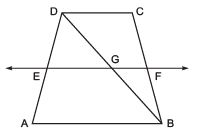Solution: In trapezium ABCD, AB || DC. E is the mid-point of AD.
EF is drawn parallel to AB. We have to prove that F is the mid-point of BC.
Join BD.
In DDAB,
∵ E is the mid-point of AD            [Given]
and EG || AB             [∵ EF || AB]
∴ Using the converse of mid-point theorem, we get that G is the mid-point BD.
Again in DBDC,
∵ G is the mid-point of BD             [Proved]
GF || DC            [∵ AB || DC and EF || AB and GF is a part of EF]
∴ Using the converse of the mid-point theorem, we get that F is the mid-point of BC.

Question 5. In a parallelogram ABCD, E and F are the mid-points of sides AB and CD respectively (see figure). Show that the line segments AF and EC trisect the diagonal BD.
Solution:
We have ABCD is a parallelogram such that: E is the mid-point of AB and F is the mid-point of CD.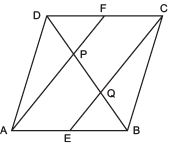Let us join the opposite vertices B and D.
Since, the opposite sides of a parallelogram are parallel and equal.
∴ AB || DC ⇒ AE || FC            …(1)

Also AB = DC
or (1/2) AB =(1/2)DC ⇒ AE = FC            …(2)
From (1) and (2),
we can say that AECF is quadrilateral having a pair of the opposite sides as parallel and equal.
∴ AEFC is a parallelogram.
⇒ AE || CF
Now, in DDBC, F is the mid-point of DC             [Given]
and FP || CQ             [∵ AF || CE]
⇒ P is the mid-point of DQ             [Converse of mid-point theorem]
⇒ DP = PQ             …(3)
Similarly, in DBAP, BQ = PQ             …(4)
∴ From (3) and (4), we have
DP = PQ = BQ
⇒ The line segments AF and EC trisect the diagonal BD.

Example 6. Show that the line segments joining the mid-points of the opposite sides of a quadrilateral bisect each other.
Solution:
A quadrilateral ABCD such that the mid-points of AB, BC, CD and DA are P, Q, R and S respectively, we have to prove that diagonals of PQRS are bisected at O.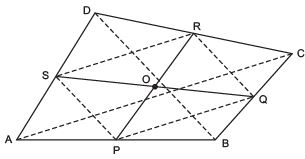Join PQ, QR, RS and SP. Let us also join PR and SQ.
Now, in ΔABC, we have P and Q as the mid-points of its sides AB and BC respectively.
∴ PQ || AC and PQ =(1/2)AC
Similarly, RS || AC and RS =(1/2)AC
⇒ PQRS is a quadrilateral having a pair of opposite sides (PQ and RS) as equal and parallel.
∴ PQRS is a parallelogram.
But the diagonals of a parallelogram bisect each other. i.e. PR and SQ bisect each other.
Thus, the line segments joining the mid-points of opposite sides of a quadrilateral ABCD bisect each other.

Question 7. ABC is a triangle, right angled at C. A line through the midpoint M of hypotenuse AB and parallel to BC intersects AC at D. Show that (i) D is the mid-point of AC (ii) MD ⊥ AC (iii) CM = MA =(1/2) AB
Solution:
We have a triangle ABC, such that ∠C = 90º M is the mid-point of AB and MD || BC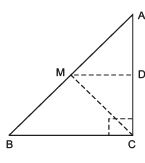(i) To prove that D is the mid-point of AC.
In ΔACB, we have M as the mid-point of AB.             [Given]
MD || BC            [Given]
∴ Using the converse of mid-point theorem, D is the mid-point of AC.

(ii) To prove that MD ⊥ AC.
Since, MD || BC             [Given] and AC is a transversal.
∴ ∠ MDA = ∠BCA            [Corresponding angles]
But ∠BCA = 90º            [Given]
∴ ∠MDA = 90º ⇒ MD ⊥ AC.

(iii) To prove that CM = MA =(1/2) AB
In ΔADM and ΔCDM, we have
∠ADM = ∠CDM            [Each = 90º]
MD = MD            [Common]
AD = CD            [∵ M is the mid-point of AC (Proved)]
∴ ΔADM ≌ ΔCOM            [SAS criteria]
⇒ MA = MC [c.p.c.t.]             …(1)
∵ M is the mid-point AB.            [Given]
∴ MA =(1/2)AB              …(2)
From (1) and (2), we have
CM = MA = (1/2) AB

,

,

,

,

,

,

,

,

,

,

,

,

,

,

,

,

,

,

,

,

,

;